Home Practice
For learners and parents For teachers and schools
Textbooks
Full catalogue
Pricing SupportLog in

We think you are located in United States. Is this correct?

# 13.3 Galvanic and electrolytic cells

## 13.3 Galvanic and electrolytic cells (ESCR3)

### Electrochemical reactions (ESCR4)

In Grade 11, you carried out an experiment to see what happens when zinc granules are added to a solution of copper(II) sulfate.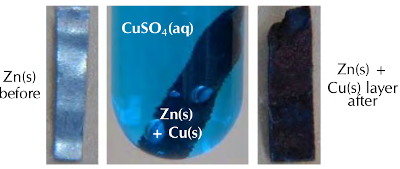Figure 13.3: When a sheet of zinc is placed in an aqueous solution of copper(II) sulfate, solid copper forms on the zinc sheet. (Screenshots taken from a video by Aaron Huggard on Youtube)

In the experiment, the $$\text{Cu}^{2+}$$ ions from the $$\color{blue}{\textbf{blue copper(II) sulfate}}$$ solution were reduced (gained electrons) to copper metal, which was then deposited as a layer on the solid zinc. The zinc atoms were oxidised (lost electrons) to form $$\text{Zn}^{2+}$$ ions in the solution. $$\text{Zn}^{2+}(\text{aq})$$ is colourless, therefore the blue solution lost colour. As discussed in Grade 11, the half-reactions are as follows:

$$\text{Cu}^{2+}(\text{aq}) + 2\text{e}^{-}$$ $$\to$$ $$\text{Cu}(\text{s})$$ ($$\color{red}{\text{reduction half-reaction}}$$)

$$\text{Zn}(\text{s})$$ $$\to$$ $$\text{Zn}^{2+}(\text{aq}) + 2\text{e}^{-}$$ ($$\color{blue}{\text{oxidation half-reaction}}$$)

The overall redox reaction is:

$$\text{Cu}^{2+}(\text{aq}) + \text{Zn}(\text{s})$$ $$\to$$ $$\text{Cu}(\text{s}) + \text{Zn}^{2+}(\text{aq})$$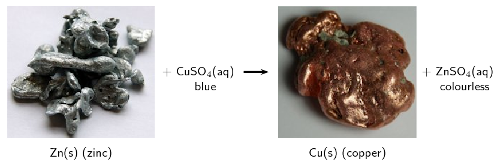Figure 13.4: Solid zinc loses two electrons to form zinc ions ($$\text{Zn}^{2+}$$) in an aqueous solution of copper(II) sulfate. The copper ions ($$\text{Cu}^{2+}$$) gain two electrons and deposit as solid copper. (Photos by benjah-bmm27 and Jurii on wikipedia)

Remember that there was an increase in the temperature of the reaction when you carried out this experiment (it was exothermic). An exothermic reaction releases energy. This raises a few questions:

• Is it possible that this heat energy could be converted into electrical energy?

• Can we use a chemical reaction with an exchange of electrons, to produce electricity?

• If we supplied an electrical current could we cause some type of chemical reaction to take place?

The answers to these questions are the focus of this chapter:

• The energy of a chemical reaction can be converted to electrical potential energy, which forms an electric current.

• The transfer of electrons in a chemical reaction can cause electrical current to flow.

• If you supply an electric current it can cause a chemical reaction to take place, by supplying the electrons (and potential energy) necessary for the reactions taking place within the cell.

These types of reactions are called electrochemical reactions. An electrochemical reaction is a reaction where:

• a chemical reaction creates an electrical potential difference, and therefore an electric current in the external conducting wires

or

• an electric current provides electrical potential energy and electrons, and therefore a chemical reaction takes place

Electrochemical reaction

An electrochemical reaction involves a transfer of electrons. There is a conversion of chemical potential energy to electrical potential energy, or electrical potential energy to chemical potential energy.

Electrochemistry is the branch of chemistry that studies these electrochemical reactions. An electrochemical cell is a device in which electrochemical reactions take place.

Electrochemical cell

A device where electrochemical reactions take place.

temp text

## Electrochemical reactions

Textbook Exercise 13.3

In each of the following equations, say which elements in the reactants are oxidised and which are reduced.

$$\text{CuO}(\text{s}) + \text{H}_{2}(\text{g})$$ $$\to$$ $$\text{Cu}(\text{s}) + \text{H}_{2}\text{O}(\text{g})$$

The oxidation number of $$\text{O}$$ is $$-\text{2}$$. $$\text{O}$$ is neither oxidised nor reduced.

The oxidation number of $$\text{Cu}$$ in:

• $$\text{CuO}(\text{s})$$ is $$\text{+2}$$

• $$\text{Cu}(\text{s})$$ is $$\text{0}$$

$$\text{Cu}^{2+}(\text{s}) + 2\text{e}^{-}$$ $$\to$$ $$\text{Cu}(\text{s})$$

$$\text{Cu}^{2+}$$ gains two electrons to form $$\text{Cu}$$. Copper is reduced

The oxidation number of $$\text{H}$$ in:

• $$\text{H}_{2}(\text{g})$$ is $$\text{0}$$

• $$\text{H}_{2}\text{O}(\text{g})$$ is $$\text{+1}$$

$$\text{H}_{2}(\text{g})$$ $$\to$$ $$2\text{H}^{+}(\text{g}) + 2\text{e}^{-}$$

$$\text{H}_{2}$$ loses two electrons to form $$2\text{H}^{+}$$. Hydrogen is oxidised.

Note: Each hydrogen atom in $$\text{H}_{2}$$ releases one electron, and these two electrons are taken up by a $$\text{Cu}^{2+}$$ ion.

$$2\text{NO}(\text{g}) + 2\text{CO}(\text{g})$$ $$\to$$ $$\text{N}_{2}(\text{g}) + 2\text{CO}_{2}(\text{g})$$

The oxidation number of $$\text{O}$$ is $$-\text{2}$$. $$\text{O}$$ is neither oxidised nor reduced.

The oxidation number of $$\text{N}$$ in:

• $$\text{NO}(\text{g})$$ is $$\text{+2}$$

• $$\text{N}_{2}(\text{g})$$ is $$\text{0}$$

$$2\text{N}^{2+}(\text{g}) + 4\text{e}^{-}$$ $$\to$$ $$\text{N}_{2}(\text{g})$$

Two $$\text{N}^{2+}$$ each gain two electrons to become $$\text{N}_{2}$$. Nitrogen is reduced.

The oxidation number of $$\text{C}$$ in:

• $$\text{CO}(\text{g})$$ is $$\text{+2}$$

• $$\text{CO}_{2}(\text{g})$$ is $$\text{+4}$$

$$\text{C}^{2+}(\text{g})$$ $$\to$$ $$\text{C}^{4+}(\text{g}) + 2\text{e}^{-}$$

$$\text{C}^{2+}$$ loses two electrons to form $$\text{C}^{4+}$$. Carbon is oxidised.

$$\text{Mg}(\text{s}) + \text{FeSO}_{4}(\text{aq})$$ $$\to$$ $$\text{MgSO}_{4}(\text{aq}) + \text{Fe}(\text{s})$$

The oxidation number of the ion $$\text{SO}_{4}$$ is $$-\text{2}$$ as both a reactant and a product.

The oxidation number of $$\text{O}$$ is $$-\text{2}$$. $$\text{O}$$ is neither oxidised nor reduced.

The oxidation number of $$\text{O}_{4}$$ in $$\text{SO}_{4}^{2-}$$ is $$-\text{8}$$. Therefore the oxidation number of $$\text{S}$$ in $$\text{SO}_{4}^{2-}$$ is $$\text{+6}$$. $$\text{S}$$ is neither oxidised nor reduced.

The oxidation number of $$\text{Mg}$$ in:

• $$\text{Mg}(\text{s})$$ is $$\text{0}$$

• $$\text{MgSO}_{4}(\text{aq})$$ is $$\text{+2}$$

$$\text{Mg}(\text{s})$$ $$\to$$ $$\text{Mg}^{2+}(\text{aq}) + 2\text{e}^{-}$$

$$\text{Mg}$$ loses two electrons to become $$\text{Mg}^{2+}$$. Magnesium is oxidised.

The oxidation number of $$\text{Fe}$$ in:

• $$\text{FeSO}_{4}(\text{aq})$$ is $$\text{+2}$$

• $$\text{Fe}(\text{s})$$ is $$\text{0}$$

$$\text{Fe}^{2+}(\text{aq}) + 2\text{e}^{-}$$ $$\to$$ $$\text{Fe}(\text{s})$$

$$\text{Fe}^{2+}$$ gains two electrons to become $$\text{Fe}$$. Iron is reduced.

$$\text{Zn}(\text{s}) + 2\text{AgNO}_{3}(\text{aq})$$ $$\to$$ $$2\text{Ag}(\text{s}) + \text{Zn}(\text{NO}_{3})_{2}(\text{aq})$$

The oxidation number of the ion $$\text{NO}_{3}$$ is $$-\text{1}$$ as both a reactant and a product.

The oxidation number of $$\text{O}$$ is $$-\text{2}$$. $$\text{O}$$ is neither oxidised nor reduced.

The oxidation number of $$\text{O}_{3}$$ in $$\text{NO}_{3}$$ is $$-\text{6}$$. Therefore the oxidation number of $$\text{N}$$ in $$\text{NO}_{3}^{-}$$ is $$\text{+5}$$. $$\text{N}$$ is neither oxidised nor reduced.

The oxidation number of $$\text{Zn}$$ in:

• $$\text{Zn}(\text{s})$$ is $$\text{0}$$

• $$\text{Zn}(\text{NO}_{3})_{2}(\text{aq})$$ is $$\text{+2}$$

$$\text{Zn}(\text{s})$$ $$\to$$ $$\text{Zn}^{2+}(\text{aq}) + 2\text{e}^{-}$$

$$\text{Zn}$$ loses two electrons to become $$\text{Zn}^{2+}$$. Zinc is oxidised.

The oxidation number of $$\text{Ag}$$ in:

• $$\text{AgNO}_{3}(\text{aq})$$ is $$\text{+1}$$

• $$\text{Ag}(\text{s})$$ is $$\text{0}$$

$$\text{Ag}^{+}(\text{aq}) + \text{e}^{-}$$ $$\to$$ $$\text{Ag}(\text{s})$$

$$\text{Ag}^{+}$$ gains an electron to form $$\text{Ag}$$. Silver is reduced.

Note: The two electrons released by Zn are taken up by two $$\text{Ag}^{+}$$ ions, this is shown in the balanced chemical equation.

Which one of the substances listed below acts as the oxidising agent in the following reaction?

$$3\text{SO}_{2}(\text{g}) + \text{Cr}_{2}\text{O}_{7}^{2-}(\text{aq}) + 2\text{H}^{+}(\text{aq})$$ $$\to$$ $$3\text{SO}_{4}^{2-}(\text{aq}) + 2\text{Cr}^{3+}(\text{aq}) + \text{H}_{2}\text{O}(\text{ℓ})$$

1. $$\text{H}^{+}$$

2. $$\text{Cr}^{3+}$$

3. $$\text{SO}_{2}$$

4. $$\text{Cr}_{2}\text{O}_{7}^{2-}$$

The oxidising agent causes another reactant to be oxidised and is itself reduced.

$$\text{H}^{+}$$ remains as $$\text{H}^{+}$$ in $$\text{H}_{2}\text{O}$$

$$\text{Cr}^{3+}$$ is a product.

$$\text{SO}_{2}$$ contains $$\text{S}^{4+}$$, while $$\text{SO}_{4}^{2-}$$ contains $$\text{S}^{6+}$$. Therefore $$\text{S}^{4+}$$ loses two electrons and is oxidised.

$$\text{Cr}_{2}\text{O}_{7}^{2-}$$ contains $$\text{Cr}^{6+}$$. Therefore $$\text{Cr}^{6+}$$ gains three electrons to become $$\text{Cr}^{3+}$$ and is reduced. It is the oxidising agent.

d) $$\text{Cr}_{2}\text{O}_{7}^{2-}$$

There are two types of electrochemical cells we will be looking more closely in this chapter: galvanic and electrolytic cells. Before we go into detail on galvanic and electrolytic cells you'll need to know a few definitions:

Electrode

An electrode is an electrical conductor that connects the electrochemical species from its solution to the external electrical circuit of the cell.

There are two types of electrodes in an electrochemical cell, the $$\color{blue}{\textbf{anode}}$$ and the $$\color{red}{\textbf{cathode}}$$.

$$\color{blue}{\text{Oxidation}}$$ always occurs at the $$\color{blue}{\textbf{anode}}$$ while $$\color{red}{\text{reduction}}$$ always occurs at the $$\color{red}{\textbf{cathode}}$$. So when trying to determine which electrode you are looking at first determine whether oxidation or reduction is occurring there. An easy way to remember this is:

Oxidation is the loss of electrons.

Reduction is the gain of electrons.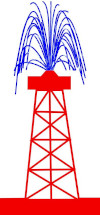$$\color{blue}{\textbf{O}}$$xidation $$\color{blue}{\textbf{i}}$$s $$\color{blue}{\textbf{l}}$$oss of electrons $$\color{blue}{\textbf{OIL}}$$ $$\color{red}{\textbf{R}}$$eduction $$\color{red}{\textbf{i}}$$s $$\color{red}{\textbf{g}}$$ain of electrons $$\color{red}{\textbf{RIG}}$$ $$\color{blue}{\textbf{O}}$$xidation is $$\color{blue}{\textbf{l}}$$oss of electrons at the $$\color{blue}{\textbf{a}}$$node $$\color{blue}{\textbf{An Ox}}$$ $$\color{red}{\textbf{Red}}$$uction is gain of electrons at the $$\color{red}{\textbf{cat}}$$hode $$\color{red}{\textbf{Red Cat}}$$

Table 13.1: A summary of phrases useful to help you remember the oxidation and reduction rules.

The electrode is placed in an electrolyte solution within the cell. If the cell is made up of two compartments, those compartments will be connected by a salt bridge.

Electrolyte

An electrolyte is a solution that contains free ions, and which therefore behaves as a conductor of charges (electrical conductor) in solution.

Salt bridge

A salt bridge is a material which contains electrolytic solution and acts as a connection between two half-cells (completes the circuit). It maintains electrical neutrality in and between the electrolytes in the half-cell compartments.

### Galvanic cells (ESCR5)

A galvanic cell (which is also sometimes referred to as a voltaic or wet cell) consists of two half-cells, which convert chemical potential energy into electrical potential energy.

Galvanic cell

A galvanic cell is an electrochemical cell which converts chemical potential energy to electrical potential energy through a spontaneous chemical reaction.

In a galvanic cell there are two half-cells. Each half-cell contains an electrode in an electrolyte. The separation is necessary to prevent direct chemical contact of the oxidation and reduction reactions, creating a potential difference. The electrons released in the oxidation reaction travel through an external circuit (and do work) before being used by the reduction reaction.

Remember that cells are not two-dimensional, although when asked to sketch a cell you should draw it as shown in Figure 13.5 or Figure 13.6.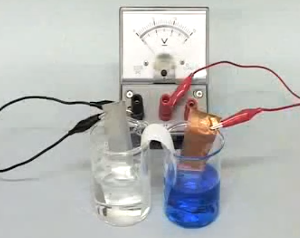In a galvanic cell (for example the cell shown in Figure 13.5):

The electrons released in the oxidation of $$\text{X}$$ remain on the anode, $$\text{X}^{+}$$ moves into solution.The $$\text{Y}^{+}$$ ions are being reduced at the cathode and forming solid $$\text{Y}$$.• The metal at the anode is $$\color{blue}{\textbf{X}}$$. $$\color{blue}{\textbf{Ox}}$$idation is loss of electrons at the $$\color{blue}{\textbf{An}}$$ode.

• The $$\color{blue}{\textbf{anode half-reaction}}$$ is $$\color{blue}{{\textbf{X(s)}} \to {\textbf{X}}^{+}{\textbf{(aq) + e}}^{-}}$$

• This half-reaction occurs in the half-cell containing the X(s) anode and the $$\text{X}^{+}(\text{aq})$$ electrolyte solution.

• The electrons released in the $$\color{blue}{\textbf{oxidation}}$$ of the metal remain on the $$\color{blue}{\textbf{anode}}$$, while the metal cations formed move into solution.

• The metal at the cathode is Y. $$\color{red}{\textbf{Red}}$$uction is gain of electrons at the $$\color{red}{\textbf{Cat}}$$hode.

• The $$\color{red}{\textbf{cathode half-reaction}}$$ is $$\color{red}{{\textbf{Y}}^{+}{\textbf{(aq) + e}}^{-} \to {\textbf{Y(s)}}}$$

• This half-reaction occurs in the half-cell containing the Y(s) cathode and the $$\text{Y}^{+}(\text{aq})$$ electrolyte solution.

• At the $$\color{red}{\textbf{cathode}}$$ metal ions in the solution are being $$\color{red}{\textbf{reduced}}$$ (accepting electrons) and deposited on the electrode.

• There are more electrons at the anode than at the cathode.

• Electrons will flow from areas of high concentration to areas of low concentration, therefore the electrons move $$\color{blue}{\textbf{from the anode}}$$, through the external circuit, $$\color{red}{\textbf{to the}}$$ $$\color{red}{\textbf{cathode}}$$

• Conventional current is measured as a flow of positive charge and so is in the opposite direction (from the cathode to the anode)

• The overall reaction is: $$\text{X}(\text{s}) + \text{Y}^{+}(\text{aq})$$ $$\to$$ $$\text{X}^{+}(\text{aq}) + \text{Y}(\text{s})$$.

• To represent this reaction using standard cell notation we write the following:

$$\color{blue}{\textbf{X(s)}}$$$$|$$$$\color{blue}{\textbf{X}^{+}{\textbf{(aq)}}}$$$$||$$$$\color{red}{\textbf{Y}^{+}{\textbf{(aq)}}}$$$$|$$$$\color{red}{\textbf{Y(s)}}$$

By convention:

• The $$\color{blue}{\textbf{anode}}$$ is always written on the $$\color{blue}{\textbf{left}}$$.

• The $$\color{red}{\textbf{cathode}}$$ is always written on the $$\color{red}{\textbf{right}}$$.

• The anode and cathode half-cells are divided by $$||$$ representing the salt bridge.

• The different phases within each half-cell (solid (s) and aqueous (aq) here) are separated by $$|$$.

• The electrodes in each half-cell are connected through a wire in the external circuit. There is also a salt bridge between the individual half-cells.

A galvanic cell uses the reactions that take place at at the two electrodes to produce electrical energy, i.e. the reaction occurs without the need to add energy.

A spontaneous reaction is one that will occur without the need for external energy. Refer to the subsection on spontaneity for more information.

The zinc-copper reaction you performed in Grade 11 can be modified to make a galvanic cell. Bars of zinc and copper are used as electrodes, with zinc(II) sulfate and copper(II) sulfate solutions as the electrolytes.

In the galvanic cell experiment, make sure that the sodium chloride paste is highly concentrated and fills the U-tube for the best results.

## A galvanic cell

### Aim

To investigate the reactions that take place in a galvanic cell.

### Apparatus

• Zinc plate, copper plate, zinc(II) sulfate ($$\text{ZnSO}_{4}$$) solution ($$\text{1}$$ $$\text{mol.dm^{-3}}$$), copper(II) sulfate ($$\text{CuSO}_{4}$$) solution ($$\text{1}$$ $$\text{mol.dm^{-3}}$$), $$\text{NaCl}$$ paste

• Measuring balance, two $$\text{250}$$ $$\text{ml}$$ beakers, U-tube, cotton wool, zero-centered ammeter, connecting wire.

• Cleaning ethanol, ether (if available)

### Method

1. Weigh the copper and zinc plates and record their mass.

2. Pour $$\text{200}$$ $$\text{ml}$$ of the zinc sulfate solution into a beaker and place the zinc plate in the beaker.

3. Pour $$\text{200}$$ $$\text{ml}$$ of the copper(II) sulfate solution into the second beaker and place the copper plate in the beaker.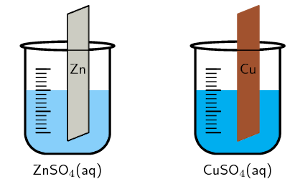4. Fill the U-tube with the $$\text{NaCl}$$ paste and seal the ends of the tubes with the cotton wool (making a salt-bridge). The cotton will help stop the paste from dissolving in the electrolyte.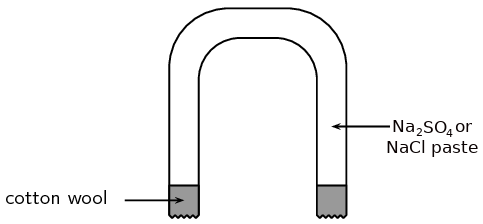5. Connect the zinc and copper plates to the zero-centered ammeter and observe the ammeter.

6. Place the U-tube so that one end is in the copper(II) sulfate solution and the other end is in the zinc sulfate solution. Observe the ammeter.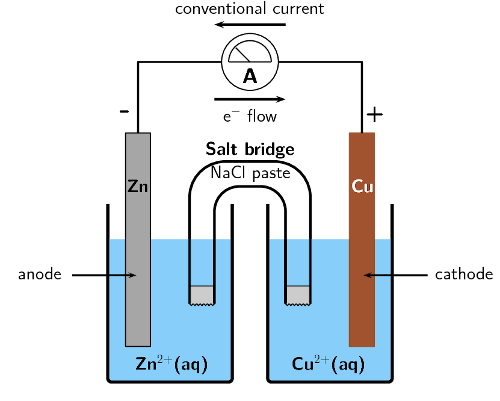7. Take the ammeter away and connect the copper and zinc plates to each other directly using copper wire. Leave to stand for about one day.

8. After a day, remove the two plates and rinse them: first with distilled water, then with alcohol, and finally with ether (if available). Dry the plates using a hair dryer.

9. Weigh the zinc and copper plates and record their mass.

### Note

A voltmeter can also be used in place of the zero-centered ammeter. A zero-centered voltmeter will measure the potential difference across the cell (not the flow of electrons), while an ammeter will measure the current.

### Discussion

• Did the ammeter record a reading before the salt-bridge was placed in the solutions?

• Did the ammeter record a reading after the salt-bridge was placed in the solutions? If yes, in what direction does the current flow?

• Fill in the table below:

 Plate Initial mass Final mass Zinc Copper
• How did the mass of the zinc and copper plates change?

• Based on what you know of oxidation and reduction, why did those mass changes take place?

• Which electrode is the anode and which is the cathode?

### Results

During the experiment, you should have noticed the following:

• When the salt bridge was absent, there was no reading on the ammeter.

• When the salt bridge was connected, a reading was recorded on the ammeter.

• The direction of electron flow is from the zinc plate towards the copper plate, meaning that conventional current flow is from the copper plate towards the zinc plate.

• After the plates had been connected directly to each other and left for a day, there was a change in their mass. The mass of the zinc plate decreased, while the mass of the copper plate increased.

• $$\color{blue}{\textbf{O}}$$xidation $$\color{blue}{\textbf{i}}$$s $$\color{blue}{\textbf{l}}$$oss of electrons, $$\color{red}{\textbf{R}}$$eduction $$\color{red}{\textbf{i}}$$s $$\color{red}{\textbf{G}}$$ain of electrons.

The zinc electrode lost mass. This implies that solid Zn metal atoms become ions and move into the electrolyte solution: $$\text{Zn}(\text{s})$$ $$\to$$ $$\text{Zn}^{2+}(\text{aq}) + 2\text{e}^{-}$$. Oxidation occurs at the zinc electrode.

The copper electrode gained mass. This implies that the Cu metal ions in the electrolyte solution become metal atoms and deposit on the electrode: $$\text{Cu}^{2+}(\text{aq}) + 2\text{e}^{-}$$ $$\to$$ $$\text{Cu}(\text{s})$$. Reduction occurs at the copper electrode.

• $$\color{blue}{\textbf{Ox}}$$idation is loss of electrons at the $$\color{blue}{\textbf{an}}$$ode. Oxidation occurs at the zinc electrode, therefore the zinc plate is the anode.

$$\color{red}{\textbf{Red}}$$uction is gain of electrons at the $$\color{red}{\textbf{cat}}$$hode. Reduction occurs at the copper electrode, therefore the copper plate is the cathode.

• When $$\text{Zn}(\text{s})$$ $$\to$$ $$\text{Zn}^{2+}(\text{aq}) + 2\text{e}^{-}$$ the electrons are deposited on the anode, which becomes negatively charged.

When $$\text{Cu}^{2+}(\text{aq}) + 2\text{e}^{-}$$ $$\to$$ $$\text{Cu}(\text{s})$$ the electrons are taken from the cathode, which becomes positively charged.

### Conclusions

When a zinc(II) sulfate solution containing a zinc plate is connected by a salt bridge to a copper(II) sulfate solution containing a copper plate, reactions occur in both solutions. The decrease in mass of the zinc plate suggests that the zinc metal electrode has been oxidised to form $$\text{Zn}^{2+}$$ ions in solution. The increase in mass of the copper plate suggests that reduction of $$\text{Cu}^{2+}$$ ions has occurred here to produce more copper metal.

The important thing to notice in this experiment is that:

• the chemical reactions that take place at the two electrodes cause an electric current to flow through the external circuit

• the overall reaction must be a spontaneous redox reaction

• chemical energy is converted to electrical energy

• the zinc-copper cell is one example of a galvanic cell

It was the Italian physician and anatomist Luigi Galvani who marked the birth of electrochemistry by making a link between chemical reactions and electricity. In 1780, Galvani discovered that when two different metals (copper and zinc for example) were connected to each other and then both touched to different parts of a nerve of a frog leg at the same time, they made the leg contract. He called this 'animal electricity'.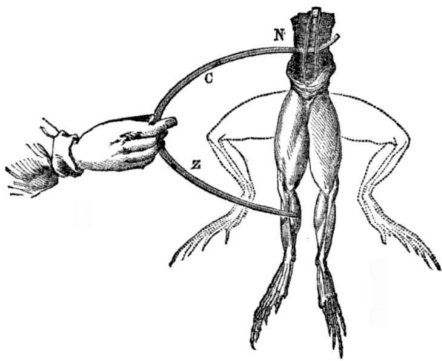In the zinc-copper cell, the copper and zinc plates are the electrodes. The salt bridge plays a very important role in a galvanic cell:

• An electrolyte solution consists of metal cations and spectator anions.

$$\text{NaCl}(\text{aq})$$ is $$\text{Na}^{+}(\text{aq})$$ and $$\text{Cl}^{-}(\text{aq})$$ in the paste.

• There is a build up of positive charge in the $$\color{blue}{\textbf{anode half-cell compartment}}$$ as solid metal is $$\color{blue}{\textbf{oxidised}}$$ and the positive ions move into solution. So there are more postive metal ions in the electrolyte than negative ions.

$$\color{blue}{\textbf{Zn(s)} \to \textbf{Zn}^{2+}\textbf{(aq)}}$$, while the number of $$\text{SO}_{4}^{2-}$$ ions remains the same.

• To balance the charge, negative ions from the salt bridge move into the anode half-cell compartment.

$$\text{Cl}^{-}$$ ions move from the salt bridge to the anode half-cell compartment.

• There is a decrease in positive charge in the $$\color{red}{\textbf{cathode half-cell compartment}}$$ as metal ions are $$\color{red}{\textbf{reduced}}$$ and form solid metal. So there are more negative ions in the electrolyte than positive metal ions.

$$\color{red}{\textbf{Cu}^{2+}\text{(aq)} \to \textbf{Cu(s)}}$$, while the number of $$\text{SO}_{4}^{2-}$$ ions remains the same.

• To balance the charge, positive ions from the salt bridge move into the cathode half-cell compartment.

$$\text{Na}^{+}$$ ions move from the salt bridge to the cathode half-cell compartment.

The salt bridge acts as a transfer medium that allows ions to flow through without allowing the different solutions to mix and react directly. It allows a balancing of the charges in the electrolyte solutions, and allows the reactions in the cell to continue.

Without the salt bridge, the flow of electrons in the outer circuit stops completely. This is because the salt bridge is needed to complete the circuit.

### Electrolytic cells (ESCR6)

In an electrolytic cell electrical potential energy is converted to chemical potential energy. An electrolytic cell uses an electric current to force a particular chemical reaction to occur, which would otherwise not take place.

Electrolytic cell

An electrolytic cell is an electrochemical cell that converts electrical potential energy to chemical potential energy by using electricity to drive a non-spontaneous chemical reaction.

Sometimes galvanic cells are just called electrochemical cells. While they are electrochemical cells, electrolytic cells are also electrochemical cells. Electrolytic and galvanic cells are not the same however.

An electrolytic cell is activated by applying an electrical potential across the electrodes to force an internal chemical reaction between the electrodes and the ions that are in the electrolyte solution. This process is called electrolysis.

Electrolysis

Electrolysis is a method of driving chemical reactions by passing an electric current through an electrolyte.

In an electrolytic cell (for example the cell shown in Figure 13.6):

• The electrolyte solution consists of the metal cations and spectator anions.

• The oxidation and reduction reactions occur in the same container but are non-spontaneous.They require the electrodes to be connected to an external power source to proceed.

• The electrodes in an electrolytic cell can be the same metal or different metals. The prinicple is the same. Let there be only one metal, and let that metal be Z.

• An electrode is connected to the $$\color{blue}{\textbf{positive terminal}}$$ of the battery.

• To balance the charge at the $$\color{blue}{\textbf{positive electrode}}$$ metal atoms are $$\color{blue}{\textbf{oxidised}}$$ to form metal ions. The ions move into solution, leaving their electrons on the electrode.

• The following reaction takes place: $$\color{blue}{\textbf{Z(s)} \to {\textbf{Z}}^{+}{\textbf{(aq)}} + {\textbf{e}}^{-}}$$

• $$\color{blue}{\textbf{Ox}}$$idation is loss at the $$\color{blue}{\textbf{an}}$$ode, therefore this electrode is the $$\color{blue}{\textbf{anode}}$$.

• An electrode is connected to the $$\color{red}{\textbf{negative terminal}}$$ of the battery.

• When positive ions come in contact with the $$\color{red}{\textbf{negative electrode}}$$ the ions gain electrons and are $$\color{red}{\textbf{reduced}}$$.

• The following reaction takes place: $$\color{red}{\textbf{Z}^{+}{\textbf{(aq)}} + {\textbf{e}}^{-} \to {\textbf{Z(s)}}}$$

• $$\color{red}{\textbf{Red}}$$uction is gain at the $$\color{red}{\textbf{cat}}$$hode, therefore this electrode is the $$\color{red}{\textbf{cathode}}$$.

• This means that the overall reaction is: $$\text{Z}(\text{s}) + \text{Z}^{+}(\text{aq})$$ $$\to$$ $$\text{Z}^{+}(\text{aq}) + \text{Z}(\text{s})$$. While this might seem trivial this is an important technique to purify metals (see Section 13.7).

In the movement of coloured ions experiment, to make the ammonia and ammonium chloride buffer solution you need to combine equimolar amounts of ammonia and ammonium chloride. The volume doesn't matter, so long as there are the same number of moles of each compound in the solution.

Concentrated, strong bases can cause serious burns. Please remind the learners to be careful and wear the appropriate safety equipment when handling all chemicals, especially strong, concentrated bases. The safety equipment includes gloves, safety glasses, and protective clothing.

## The movement of coloured ions under the effect of electrical charge

### Aim

To demonstrate how ions migrate in solution towards oppositely charged electrodes.

### Apparatus

• Filter paper, glass slide, a 9V battery, two crocodile clips connected to wires, tape

• Ammonia ($$\text{NH}_{3}(\text{aq})$$) and ammonium chloride ($$\text{NH}_{4}\text{Cl}$$) buffer solution, copper(II) chromate solution

### Method

1. Connect the wire from one crocodile clip to one end of the battery and secure with tape. Repeat with the other crocodile clip wire and the other end of the battery.

2. Soak a piece of filter paper in the ammonia and ammonium chloride buffer solution and place it on the glass slide.

3. Connect the filter paper to the battery using one of the crocodile clips, keep the other one nearby.

4. Place a line of copper(II) chromate solution at the centre of the filter paper. The colour of this solution is initially green-brown.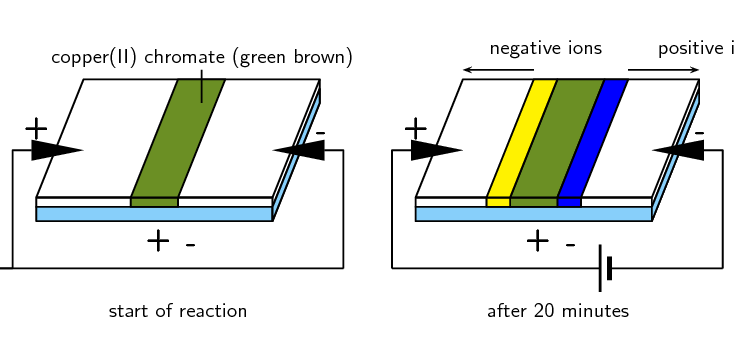5. Attach the other crocodile clip opposite the first one (as shown in the diagram) and leave the experiment to run for about $$\text{20}$$ $$\text{minutes}$$.

### Results

• After $$\text{20}$$ $$\text{minutes}$$ you should see that the central coloured band disappears and is replaced by two bands, one yellow and the other blue, which seem to have separated out from the first band of copper(II) chromate.

• The cell that is used to supply an electric current sets up a potential difference across the circuit, so that one of the electrodes is positive and the other is negative.

• The chromate ($$\text{CrO}_{4}^{2-}$$) ions in the copper(II) chromate solution are attracted to the positive electrode, this creates a yellow band. The $$\text{Cu}^{2+}$$ ions are attracted to the negative electrode, this creates a blue band.

### Conclusion

The movement of ions occurs because the electric current in the external circuit provides a potential difference between the two electrodes.

In the electrolytic cell experiment it is important that the learners weigh the copper electrodes carefully before the experiment, and wash and dry the electrodes before weighing after the experiment.

## An electrolytic cell

### Aim

To investigate the reactions that take place in an electrolytic cell.

### Apparatus

• Two copper plates (of equal size and mass), copper(II) sulfate ($$\text{CuSO}_{4}$$) solution ($$\text{1}$$ $$\text{mol.dm^{-3}}$$)

• A $$\text{9}$$ $$\text{V}$$ battery, two connecting wires, a beaker.

### Method

1. Half fill the beaker with copper(II) sulfate solution. What colour is the solution?

2. Weigh each copper electrode carefully and record the weight.

3. Place the two copper electrodes (of known mass) in the solution and make sure they are not touching each other.

4. Connect the electrodes to the battery as shown below and leave the experiment for a day. What colour is the solution after a day?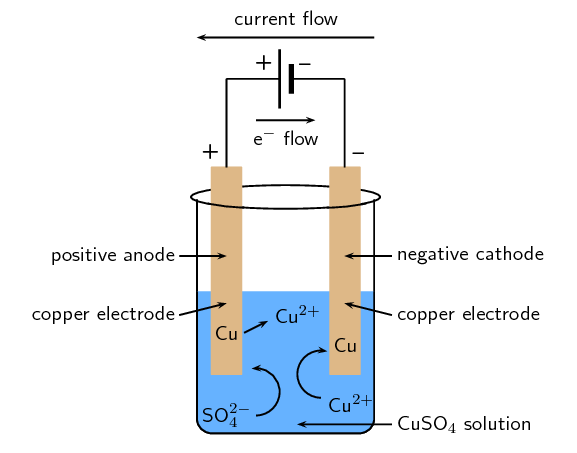### Discussion

• What colour was the copper(II) sulfate solution before the experiment?

• What colour was the copper(II) sulfate solution after the experiment?

• Examine the two electrodes, what do you observe?

• What is the charge on each electrode?

• Which electrode is the anode and which is the cathode?

### Observations

• The initial blue colour of the solution remains unchanged throughout the experiment.

• It appears that copper has been deposited on one of the electrodes (it increased in mass) but dissolved from the other (it decreased in mass).

• The electrode connected to the negative terminal of the battery will have a negative charge. The electrode connected to the positive terminal of the battery will have a positive charge.

• When positively charged $$\text{Cu}^{2+}$$ ions encounter the negatively charged electrode they gain electrons and are reduced to form copper metal. This metal is deposited on the electrode. The half-reaction that takes place is as follows:

$$\text{Cu}^{2+}(\text{aq}) + 2\text{e}^{-}$$ $$\to$$ $$\text{Cu}(\text{s})$$ (reduction half-reaction)

Reduction occurs at the cathode. Therefore, the electrode which increased in mass is the cathode.

• At the positive electrode, copper metal is oxidised to form $$\text{Cu}^{2+}$$ ions, leaving electrons on the electrode. The half-reaction that takes place is as follows:

$$\text{Cu}(\text{s})$$ $$\to$$ $$\text{Cu}^{2+}(\text{aq}) + 2\text{e}^{-}$$ (oxidation half-reaction)

Oxidation occurs at the anode. Therefore, the electrode which decreased in mass is the anode.

• The amount of copper that is deposited at one electrode is approximately the same as the amount of copper that is dissolved from the other. The number of $$\text{Cu}^{2+}$$ ions in the solution therefore remains almost the same, and the blue colour of the solution is unchanged.

### Conclusion

In this demonstration, the container held aqueous $$\text{CuSO}_{4}$$ ($$\text{Cu}^{2+}(\text{aq})$$ and $$\text{SO}_{4}^{2-}(\text{aq})$$). The copper atoms of the electrode connected to the positive terminal (the anode) were oxidised and formed $$\text{Cu}^{2+}(\text{aq})$$ ions, causing a decrease in mass. The copper atoms of the electrode connected to the negative terminal (the cathode) were reduced to form solid copper, causing an increase in mass. This process is called electrolysis, and is very useful in the purification of metals.

Note that the cathode is negative and the anode is positive. Reduction still occurs at the cathode (Red Cat), and oxidation still occurs at the anode (An Ox).The electrolysis of water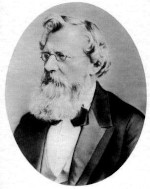August Wilhelm von Hofmann was a German chemist who invented the Hofmann cell, which uses a current to form $$\text{H}_{2}(\text{g})$$ and $$\text{O}_{2}(\text{g})$$ from water through electrolysis.

Water can undergo electrolysis to form hydrogen gas and oxygen gas according to the following reaction:

$$2\text{H}_{2}\text{O}(\text{ℓ})$$ $$\to$$ $$2\text{H}_{2}(\text{g}) + \text{O}_{2}(\text{g})$$

This reaction is very important because hydrogen gas has the potential to be used as an energy source. The electrolytic cell for this reaction consists of two electrodes, submerged in an electrolyte and connected to a source of electric current (Figure 13.7).

The $$\color{blue}{\textbf{oxidation half-reaction}}$$ is as follows: The $$\color{red}{\textbf{reduction half-reaction}}$$ is as follows:

$$\color{blue}{\text{2H}_{2}\text{O(l)} \to \text{O}_{2}\text{(g) + 4H}^{+}\text{(aq) + 4e}^{-}} \color{red}{\text{ 2H}^{+}\text{(aq) + 2e}^{-} \to \text{H}_{2}\text{(g)}}$$

The informal experiment is the electrolysis of sodium iodide and water. It would be advisable to check that current flows through the pencils, as the pencil lead may be broken. If you have access to graphite rods they can be used instead. In the second part of the experiment, there should be an obvious colour change when the phenolphthalein and $$\text{NaOH}$$ mix at the cathode.

Learners are required to work with a concentrated, strong acid. Concentrated, strong acids can cause serious burns. Please remind the learners to be careful and wear the appropriate safety equipment when handling all chemicals, especially concentrated acids. The safety equipment includes gloves, safety glasses and protective clothing.

temp text

## The electrolysis of sodium iodide and water

### Aim

To study the electrolysis of water and sodium iodide.

### Apparatus

• $$\text{4}$$ pencils, copper wire attached to crocodile clips, $$\text{9}$$ $$\text{V}$$ battery, $$\text{2}$$ beakers, pencil sharpener, spatula, glass rod

• distilled water, sodium iodide ($$\text{NaI}$$), phenolphthalein, $$\text{1}$$ $$\text{mol.dm^{-3}}$$ sulfuric acid ($$\text{H}_{2}\text{SO}_{4}$$) solution

### Method

1. Label one beaker 1 and half fill it with distilled $$\text{H}_{2}\text{O}$$.2. Sharpen both ends of two of the pencils. Strip away some of the wood to expose more of the graphite. (Graphite rods can be used instead of pencils if they are available).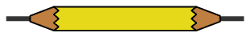3. Attach one end of the crocodile clips to the pencils and the other end to the battery.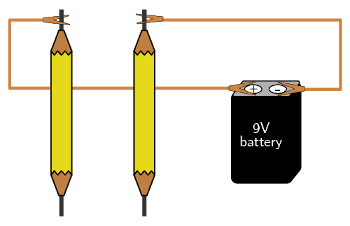4. Place the pencils in the beaker, making sure they are not touching each other. Observe what happens.

5. Pour approximately $$\text{5}$$ $$\text{cm^{3}}$$ of the $$\text{H}_{2}\text{SO}_{4}$$ solution into the beaker. Observe what happens.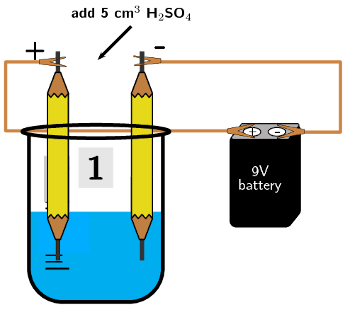6. Label a second beaker 2 and add $$\text{5}$$ spatula tips of $$\text{NaI}$$ to the beaker.

7. Half fill beaker $$\text{2}$$ with distilled $$\text{H}_{2}\text{O}$$ and stir with the glass rod until all the sodium iodide has dissolved.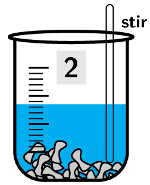8. Repeat steps $$\text{2}$$ - $$\text{4}$$ with the second set of pencils.

9. After a few minutes add $$\text{3}$$ - $$\text{4}$$ drops of the phenolphthalein to the beaker. Observe what happens.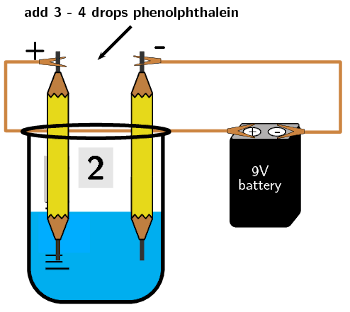### Questions

• In beaker 1:

1. What happened when the pencils were first put in the water?

2. What happened when the sulfuric acid was added to the beaker?

3. Why is the sulfuric acid necessary in this reaction?

4. What is happening at the negative electrode (the pencil attached to the negative terminal of the battery)?

5. What is happening at the positive electrode (the pencil attached to the positive terminal of the battery)?

6. Which electrode is the anode and which is the cathode?

• In beaker 2:

1. What happened when the pencils were first put in the water?

2. What is happening at the negative electrode?

3. What is happening at the positive eletrode?

4. Which electrode is the anode and which is the cathode?

5. What happened when you added the phenolphthalein? Why did the change take place?

### Results

• In the electrolysis of water two $$\text{H}^{+}$$ ions each gain an electron (are reduced) and combine to form hydrogen gas ($$\text{H}_{2}(\text{g})$$):

$$2\text{H}^{+}(\text{aq}) + 2\text{e}^{-}$$ $$\to$$ $$\text{H}_{2}(\text{g})$$

Two $$\text{O}^{2-}$$ ions each lose two electrons (are oxidised) and combine to form oxygen gas ($$\text{O}_{2}(\text{g})$$):

$$2\text{O}^{2-}(\text{aq})$$ $$\to$$ $$\text{O}_{2}(\text{g}) + 4\text{e}^{-}$$

• When the positive $$\text{H}^{+}$$ ions encounter the negative electrode they are reduced. Reduction is a gain of electrons at the cathode, therefore the negative electrode is the cathode.

• When the negative $$\text{O}^{2-}$$ ions encounter the positive electrode they are oxidised. Oxidation is a loss of electrons at the anode, therefore the positive electrode is the anode.

• Remember that pure water does not conduct electricity. So, an electrolyte (such as sulfuric acid) is necessary for the reaction to take place.

• A salt dissolved in the water is also an electrolyte, so sulfuric acid is not necessary in the second beaker.

• The electrolysis of water occurs in beaker 2, but there are other reactions taking place as well because of the presence of the $$\text{Na}^{+}$$ and $$\text{I}^{-}$$ ions.

• When the negative $$\text{I}^{-}$$ ions encounter the positive electrode they are oxidised:

$$2\text{I}^{-}(\text{aq})$$ $$\to$$ $$\text{I}_{2}(\text{s}) + 2\text{e}^{-}$$

You should have observed solid iodine forming at the positive electrode.

• When the positive $$\text{Na}^{+}$$ ions encounter the negative electrode they are reduced:

$$\text{Na}^{+}(\text{aq}) + \text{e}^{-}$$ $$\to$$ $$\text{Na}(\text{s})$$

$$\text{Na}(\text{s})$$ is very reactive with water:

$$2\text{Na}(\text{s}) + 2\text{H}_{2}\text{O}(\text{ℓ})$$ $$\to$$ $$2\text{NaOH}(\text{aq}) + \text{H}_{2}(\text{g})$$.

• Remember that phenolphthalein turns pink in the presence of a base. So when the phenolphthalein is added, the water around the negative electrode should become pink due to the $$\text{NaOH}$$.

### Conclusion

The application of an electric current to water splits the water molecules and causes hydrogen and oxygen gas to form. This can only happen in the presence of an electrolyte (for example sulfuric acid). Sodium iodide dissolved in the water is also an electrolyte and enables the electrolysis of water. However, the cation and anion of the salt will also undergo a reaction. $$\text{NaOH}$$ will form at the cathode, while solid iodine will form at the anode.

## Galvanic and electrolytic cells

Textbook Exercise 13.4

An electrolytic cell consists of two electrodes in a silver chloride ($$\text{AgCl}$$) solution, connected to a source of current. A current is passed through the solution and $$\text{Ag}^{+}$$ ions are reduced to a silver metal deposit on one of the electrodes.

What is the name of this process?

Electrolysis

Does reduction occur at the electrode where the deposit formed?

Yes. Reduction is a gain of electrons. In an electrolytic cell the electrode connected to the negative terminal of the battery is negative. When the metal cations encounter this electrode they gain electrons and form metal atoms that deposit on the electrode ($$\text{Ag}^{+}(\text{aq}) +\text{e}^{-}$$ $$\to$$ $$\text{Ag}(\text{s})$$).

Give the equation for the reduction half-reaction.

$$\text{Ag}^{+}(\text{aq}) + \text{e}^{-}$$ $$\to$$ $$\text{Ag}(\text{s})$$

Give the equation for the oxidation half-reaction.

$$\text{Ag}(\text{s})$$ $$\to$$ $$\text{Ag}^{+}(\text{aq}) + \text{e}^{-}$$

A galvanic cell consists of two half-cells: a copper anode in a copper nitrate ($$\text{Cu}(\text{NO}_{3})_{2}(\text{aq})$$) solution, and a silver cathode in a silver nitrate ($$\text{AgNO}_{3}(\text{aq})$$) solution.

Give equations for the half-reactions that take place at the anode and cathode.

You are told that the copper electrode is the anode, oxidation is a loss of electrons at the anode:

Anode half-reaction: $$\text{Cu}(\text{s})$$ $$\to$$ $$\text{Cu}^{2+}(\text{aq}) + 2\text{e}^{-}$$

You are told that the silver electrode is the cathode, reduction is a gain of electrons at the cathode:

Cathode half-reaction: $$\text{Ag}^{+}(\text{aq}) + \text{e}^{-}$$ $$\to$$ $$\text{Ag}(\text{s})$$

Write the overall reaction for this cell.

$$\text{Cu}(\text{s}) + 2\text{Ag}^{+}(\text{aq})$$ $$\to$$ $$\text{Cu}^{2+}(\text{aq}) + 2\text{Ag}(\text{s})$$

OR

$$\text{Cu}(\text{s}) + 2\text{AgNO}_{3}(\text{aq})$$ $$\to$$ $$\text{Cu}(\text{NO}_{3})_{2}(\text{aq}) + 2\text{Ag}(\text{s})$$

Give standard cell notation for this cell.

$$\text{Cu}(\text{s})|\text{Cu}^{2+}(\text{aq})||\text{Ag}^{+}(\text{aq})|\text{Ag}(\text{s})$$

Draw a simple diagram of the galvanic cell.

On your diagram, show the direction in which current flows.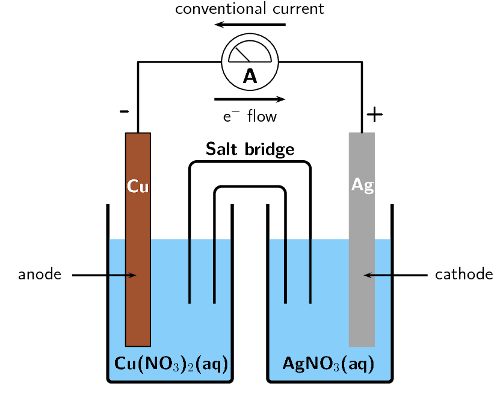Remember that conventional current flow is in the opposite direction to electron flow.

Electrolysis takes place in a solution of molten lead bromide ($$\text{PbBr}_{2}$$) to produce lead atoms.

Give equations for the half-reactions that take place at the anode and cathode.

Oxidation is a loss of electrons at the anode:

Anode half-reaction: $$\text{Pb}(\text{s})$$ $$\to$$ $$\text{Pb}^{2+}(\text{ℓ}) + 2\text{e}^{-}$$

Reduction is a gain of electrons at the cathode:

Cathode half-reaction: $$\text{Pb}^{2+}(\text{ℓ}) + 2\text{e}^{-}$$ $$\to$$ $$\text{Pb}(\text{s})$$

Draw a simple diagram of the electrolytic cell.

On your diagram, show the direction in which current flows.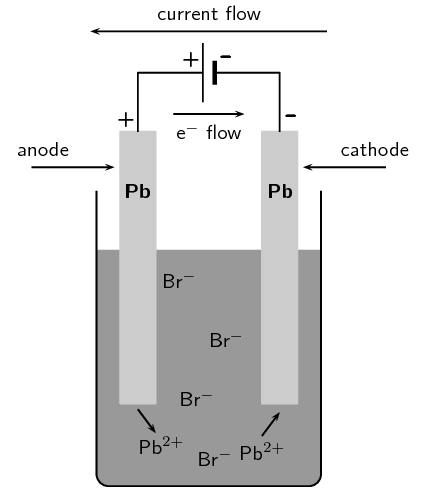Remember that conventional current flow is in the opposite direction to electron flow.

Fill in the table below to summarise the information on galvanic and electrolytic cells:

 Galvanic cells Electrolytic cells spontaneity type of energy anode cathode cell set-up
 Galvanic cells Electrolytic cells spontaneity spontaneous reactions non-spontaneous reactions type of energy converts chemical potential energy to electrical energy converts electrical energy to chemical potential energy anode is negative, oxidation occurs at anode is positive, oxidation occurs at anode cathode is positive, reduction occurs at cathode is negative, reduction occurs at cathode cell set-up two half-cells, one electrode in each, connected by a salt-bridge one cell, both electrodes in cell, no salt-bridge